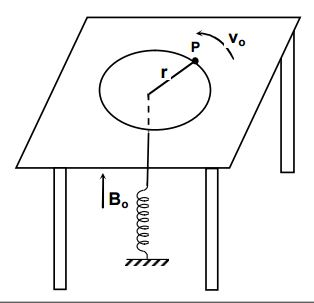# Magnetic Circular Motion!

A particle mass $m$ charge $q$ is moving on a circular path on the surface of a friction less table with speed $v_o$ where a magnetic field $B_o$ exists uniformly over the whole region. It is attached by a string which passes through a hole in the table to a spring as shown. The spring is stretched by $x_o$. If now the magnetic field is increased slowly to $2B_o$,

(1) The extension in the spring will increase.

(2) The speed of the particle will increase.

(3) The speed of the particle will decrease.

(4) The kinetic energy of the particle will decrease.Note - If your answer is $(1,2)$, Please mark your answer as $3 (=1+2)$

×